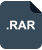### matlab调用C++ 动态库实例 评分:

2018-11-07 上传大小：136KBc++ 调用matlab 动态链接库Matlab调用C++代码的具体mex实现详解MATLAB调用C++程序Matlab中调用C++dllMATLAB调用C/C++函数的方法matlab调用c++函数matlab中调用c++函数MATLAB与C/C++混合编程之MATLAB调用C程序MATLAB编译并调用C、C++程序 立即下载matlab调用c++ 立即下载matlab调用c++生成的DLLc++调用matlab生成的Dll动态连接库 立即下载C++与Matlab混编，调用生成的动态链接库MATLAB调用C/C++编写的文件,例 .dll .mexw64等matlab调用VS生成的DLL【转】matlab 生成调用c/c++ 代码 mex 简单实用——修改部分matlab可以调用c++的DLL吗？C++中如何调用自己创建的动态链接库中的类及相关的函数，麻烦详细说明下VC与Matlab混合编程之调用动态链接库dll(C++)——<二>Matlab调用c++闪退问题csdn_now### 热点文章

• #### c++ 调用matlab 动态链接库

2014-09-20 sduvec
• #### Matlab调用C++代码的具体mex实现详解

2017-11-21 sinat_38321889
• #### MATLAB调用C++程序

2018-01-15 john_bian
• #### Matlab中调用C++dll

2018-05-10 z215367701
• #### MATLAB调用C/C++函数的方法

2013-01-10 camby747
• #### matlab调用c++函数

2017-01-17 Ben_Ben_Niao
• #### matlab中调用c++函数

2017-04-12 m0_37407756
• #### MATLAB与C/C++混合编程之MATLAB调用C程序

2017-04-09 Eric2016_Lv
• #### MATLAB编译并调用C、C++程序

2016-06-06 xidiancoder

#### 公告### spring mvc+mybatis+mysql+maven+bootstrap 整合实现增删查改简单实例.zip

 资源所需积分/C币 当前拥有积分 当前拥有C币 5 0 0VIP下载

## 积分不足！

 资源所需积分/C币 当前拥有积分

 4000万 程序员的必选 600万 绿色安全资源 现在开通 立省522元资源所需积分/C币 当前拥有积分 当前拥有C币 5 4 45资源所需积分/C币 当前拥有积分 当前拥有C币 5 0 0资源所需积分/C币 当前拥有积分 当前拥有C币 5 4 45

• 举报人：
• 被举报人：
• *类型：
• *投诉人姓名：
• *投诉人联系方式：
• *版权证明：
• *详细原因：